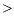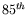Computational & Technology Resources an online resource for computational,engineering & technology publications not logged in - login Civil-Comp ProceedingsISSN 1759-3433 CCP: 80PROCEEDINGS OF THE FOURTH INTERNATIONAL CONFERENCE ON ENGINEERING COMPUTATIONAL TECHNOLOGY Edited by: B.H.V. Topping and C.A. Mota Soares Paper 29Stochastic Stability of Fluid-Structure Coupled Systems with Multiplicative and Additive Noise: Application to Airfoil Flutter I. Zentner+* and F. Poirion++French Aeronautics and Space Research Center (ONERA), Paris, France *National Superior School of Civil Engineering (ENPC), Paris, France doi:10.4203/ccp.80.29 Full Bibliographic Reference for this paper I. Zentner, F. Poirion, "Stochastic Stability of Fluid-Structure Coupled Systems with Multiplicative and Additive Noise: Application to Airfoil Flutter", in B.H.V. Topping, C.A. Mota Soares, (Editors), "Proceedings of the Fourth International Conference on Engineering Computational Technology", Civil-Comp Press, Stirlingshire, UK, Paper 29, 2004. doi:10.4203/ccp.80.29 Keywords: aeroelasticity, random dynamical system, stochastic stability, stochastic bifurcation, Lyapunov exponent, flutter instability, limit cycle oscillation. Summary This paper is concerned with the investigation of stability of fluid-structure coupled systems in turbulent flow. The field of turbulence is modeled by stationary stochastic processes and may introduce multiplicative as well as additive noise in the original deterministic formulation. The existence of an ergodic invariant measure (typically the Dirac measure for a fixed point) for the random dynamical systems thus obtained allows for a local analysis of the flow based on the multiplicative ergodic theorem of Oseledets, which provides a substitute of certain features of linear algebra. The Lyapunov exponents give the exponential expansion rates for the linear (or linearized) system and generalize thus the notion of the real parts of eigenvalues. The corresponding linear subspaces where these expansion rates are realized are called the Oseledets spaces. If the largest Lyapunov exponent is negative, the system will be stable with probability one. Robust methods for numerical analysis of deterministic bifurcation problems are available (see for example Doedel). Stochastic bifurcation theory, however, is still in its beginning . Insight in the behavior of complex systems (dimension2) has been obtained only by numerical simulation so far. For our purpose, we adopt the bifurcation criterion recently introduced by Arnold et al., that is the change of sign of the largest Lyapunov exponent. Numerous case studies concerning the asymptotic behavior of the noisy Duffing van der Pol oscillator have been published in the last years by mathematicians as well as engineers. Our aim is to apply this rather recent theory to high dimensional complex problems as they occur in aeronautical engineering. As for the two-dimensional airfoil, the aerodynamic forces are determined in the frequency domain by solving the linearized Euler equations. In this formulation, the non-stationary velocity field is decomposed into a stationary part and its first order perturbation . It is well known that a linear airfoil subjected to a fluid flow (no turbulence) may exhibit flutter instability for a certain flow velocity. Taking into account a structural freeplay non-linearity, the phenomenon is modified and there is a Hopf bifurcation and limit cycle oscillations . We propose to study the impact of noise i.e. (atmospheric) turbulence on these phenomena where the spectral density of the turbulence is supposed to be known (we use the von Karman model). These spectra can be approximated by rational fractions using, for instance, the method of simulated annealing. The trajectories of the stochastic processes representing longitudinal and vertical turbulence can then be obtained as solutions of stochastic differential equations SDE (method of Markovianisation ). The high-dimensionality (a dozen degrees of freedom for the two-dimensional problem and many more for the complete 3D problem) as well as the complexity of the problems treated make it impossible to obtain analytical results but require numerical simulation. The constitutive equations of the coupled system may be written either in the form of SDE or as what are called random differential equations (excited by real noise)  . The latter can be considered as non-autonomous ordinary differential equations if the noise is sufficiently smooth and thus allow for numerical simulation by classical schemes. The advantages and the accuracy of both stochastic and classical numerical schemes are investigated. The results obtained for the two-dimensional airfoil subjected to turbulent flow will be presented. References 1 Arnold, L. "Random dynamical systems", Springer Verlag, Berlin Heidelberg, 1998. 2 Arnold, L. "Recent progress in stochastic bifurcation theory", In: IUTAM Symposium on Nonlinearity and Stochastic Structural Dynamics 1999, Madras India, 15-27, edited by S.Narayanan and R.N.Iyengar, Kluwer Dordrecht, 2001. 3 Dimitrijevic, Z., Mortchelewicz G., Poirion, F., "Nonlinear dynamics of a two dimensional airfoil with freeplay in an inviscid compressible flow", Aerospace Science and Technology, 4, 125-133, 2000. doi:10.1016/S1270-9638(00)00127-9 4 Kloeden, P.E., Platen, E., "Numerical solution of stochastic differential equations", Springer Verlag, Berlin Heidelberg, 1992. 5 Kree, P., Soize, C., "Mathematics of random phenomena", Reidel Publishing Company, Dordrecht, Holland, 1986. 6 Mortchelewicz, G.D., "Application of the linearized Euler equations to flutter",Agard SMP Meeting, Aalborg, Denmark, 1997. 7 Poirion, F., "On some stochastic methods applied to aeroservoelasticity", Aerospace Science and Technology, 4, 201-214, 2000. doi:10.1016/S1270-9638(00)00118-8 8 Talay, D., "Approximation of upper Lyapunov exponents of bilinear stochastic differential systems", SIAM Journal of Numerical Analysis, Vol.28, 4, 1141-1164, 1991. doi:10.1137/0728061 purchase the full-text of this paper (price £20) Back to top ©Civil-Comp Limited 2020 - terms & conditions